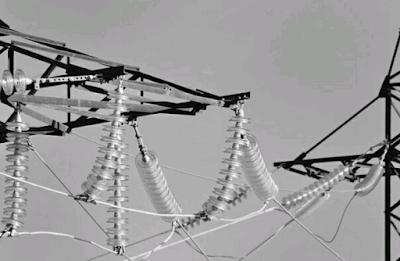### Ticker

6/recent/ticker-posts

# PERFORMANCE OF SHORT AND MEDIUM TRANSMISSION LINES

## Short And Medium Transmission lines

The important Consideration in the operation of the transmission lines are voltage drop and power losses occuring in the line and Efficiency of Transmission.

These are largely influenced by the transmission line Constants i.e., Resistance R, Inductance L, and capacitance C.Electrical Transmission line

The Resistance R is due to the fact that every Conductors offers opposition to the flow of current.

The Inductance L is due to the fact that Conductor is surrounded by the Magnetic lines of force.

The Capacitance C of the line is due to fact that the conductor carrying Current forms a capacitor with the Earth which is always at lower potential than the conductor and air between them forms a Dielectric medium.

The effect of line resistance is to cause voltage drop IR Volts and Power loss I²R watts in the line.

The effect of line Inductance is to cause voltage drop in the line in quadrature with the current flowing in the Conductor, which is equal to 2πfLI Volts where f is supply frequency in Hz, L is the Inductance per Conductor in henries, I is the Current in Ampere.

The effect of the line capacitance is to Produce a current, called the charging Current.

Which is in quadrature with the voltage. This component of current in the Conductor has its maximum value at the sending end of the line and decreases continuously as the receiving end of the line is approached at Which point its value is Zero.

#The magnitude of charging Current at the sending end is given by 2πfCVs.Power Supply line

Where f is the supply frequency in Hz, C is Capacitance per Conductor in Farads, Vs is the Voltage ( at sending end ) to neutral in Volts.

It is noteworthy that both the Reactive drop ( 2πfLI ) and the charging Current ( 2πfCVs )
Of the line are Proportional to supply frequency and thus have a far greater influence on the performance of a 50 Hz line than on a 25 Hz line.

Also that with overhead line Reactance effects are relatively more important owing to wide spacing of the Conductors which must be employed, whilst in the case of Transmission by Underground Cables Reactance effects are small and the Capacitance effect predominates.

### Classification of Transmission lines

Generally Transmission lines are classified in to Short, medium and long Transmission lines.

Transmission lines having length lesser than 60 km and operating at voltage lower than 20,000 volts fall in first catagory
i.e., Short Transmission lines. Due to smaller distance and lower line Voltage, the Capacitance effects are extremely small and, there fore can be neglected.

Hence the performance of Short Transmission lines depends upon the resistance and capacitance of the line.

Through in actual line, the resistance and Inductance and Distributed along the whole length, but in case of Short lines, the Total resistance and capacitance are assumed to be lumped at one place.

In case single phase Circuits, the Total loop resistance and Inductance is to be taken into account, whereas, in case of 3 - phase Circuits only resistance and Inductance to neutral i.e., per phase is required to be taken into account.

The effects of Generators and Transformers can be Represented by the series line Impedance, line the Synchronous Impedance of the Generator and the series Impedance of the Transformer.